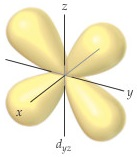# Problem: The accompanying drawing shows a contour plot for a dyz orbital. Consider the quantum numbers that could potentially correspond to this orbital.The probability density goes to zero along which of the following planes: xy, xz, or yz?

###### FREE Expert Solution

We have to determine the plane at which the electron probability density goes to zero for a dyz orbtail.

The regions of an orbital where electrons are found are called its lobes.

100% (389 ratings)###### Problem Details

The accompanying drawing shows a contour plot for a dyz orbital. Consider the quantum numbers that could potentially correspond to this orbital.The probability density goes to zero along which of the following planes: xy, xz, or yz?

Frequently Asked Questions

What scientific concept do you need to know in order to solve this problem?

Our tutors have indicated that to solve this problem you will need to apply the Quantum Numbers: Nodes concept. You can view video lessons to learn Quantum Numbers: Nodes. Or if you need more Quantum Numbers: Nodes practice, you can also practice Quantum Numbers: Nodes practice problems.

What professor is this problem relevant for?

Based on our data, we think this problem is relevant for Professor Hurst's class at LSU.